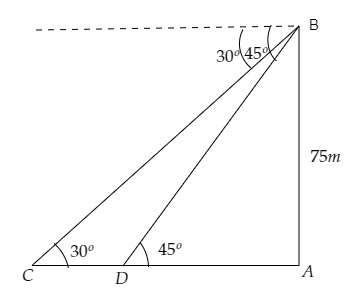# As observed from the top of a $75\ m$ high lighthouse from the sea-level, the angles of depression of two ships are $30^o$ and $45^o$. If one ship is exactly behind the other on the same side of the lighthouse, find the distance between the two ships.

Given:

As observed from the top of a $75 \mathrm{~m}$ tall lighthouse, the angles of depression of two ships are $30^{\circ}$ and $45^{\circ}$.

One ship is exactly behind the other on the same side of the lighthouse.

To do:

We have to find the distance between the two ships.

Solution:Let $AB$ be the height of the tall lighthouse and $C, D$ are the two ships one behind the other.

From the figure,

$\mathrm{AB}=75 \mathrm{~m}, \angle \mathrm{BCA}=30^{\circ}, \angle \mathrm{BDA}=45^{\circ}$

Let the distance between ship $D$ and the lighthouse be $\mathrm{DA}=x \mathrm{~m}$ and the distance between the two ships be $\mathrm{CD}=y \mathrm{~m}$.

This implies,

$\mathrm{CA}=x+y \mathrm{~m}$

We know that,

$\tan \theta=\frac{\text { Opposite }}{\text { Adjacent }}$

$=\frac{\text { AB }}{DA}$

$\Rightarrow \tan 45^{\circ}=\frac{75}{x}$

$\Rightarrow 1=\frac{75}{x}$

$\Rightarrow x=75 \mathrm{~m}$.........(i)

Similarly,

$\tan \theta=\frac{\text { Opposite }}{\text { Adjacent }}$

$=\frac{\text { BA }}{CA}$

$\Rightarrow \tan 30^{\circ}=\frac{75}{x+y}$

$\Rightarrow \frac{1}{\sqrt3}=\frac{75}{75+y}$              [From (i)]

$\Rightarrow 75+y=75\sqrt3 \mathrm{~m}$

$\Rightarrow y=75(\sqrt3-1) \mathrm{~m}$

Therefore, the distance between the two ships is $75(\sqrt3-1) \mathrm{~m}$.

Updated on: 10-Oct-2022

36 Views